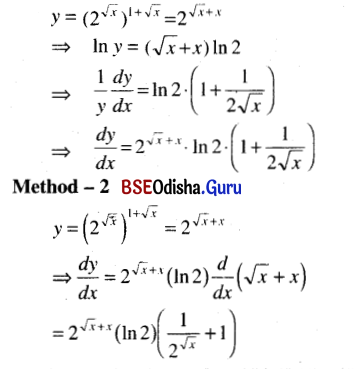# CHSE Odisha Class 12 Math Solutions Chapter 7 Continuity and Differentiability Ex 7(f)

Odisha State Board Elements of Mathematics Class 12 CHSE Odisha Solutions Chapter 7 Continuity and Differentiability Ex 7(f) Textbook Exercise Questions and Answers.

## CHSE Odisha Class 12 Math Solutions Chapter 7 Continuity and Differentiability Exercise 7(f)

Find derivatives of the following functions.
Question 1.
xx
Solution:
Let y = xx
Then In y = x . In x
⇒ $$\frac{d}{d x}$$(In y) = $$\frac{d}{d x}$$(x . In x)
⇒ $$\frac{1}{y}$$$$\frac{d y}{d x}$$ = In x + x . $$\frac{1}{x}$$ = In x + 1 = 1 + In x
⇒ $$\frac{d y}{d x}$$ = y (1 + In x) = xx (1 + In x) = xx In (ex)

Question 2.
$$\left(1+\frac{1}{x}\right)^x$$
Solution: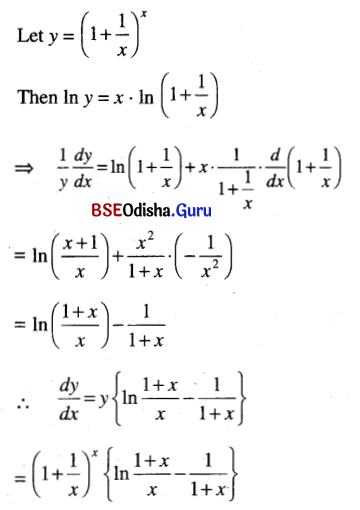Question 3.
xsin x
Solution:
y = xsin x
⇒ In y = sin x . In x
⇒ $$\frac{1}{y}$$$$\frac{d y}{d x}$$ = cos x In x + sin x . $$\frac{1}{x}$$
⇒ $$\frac{d y}{d x}$$ = xsin x (cos x . In x + $$\frac{\sin x}{x}$$)

Question 4.
(log x)tan x
Solution:
y = (log x)tan x
⇒ log y = tan x . log (log x)
⇒ $$\frac{1}{y}$$$$\frac{d y}{d x}$$ = sec2 x log (log x) + tan x . $$\frac{1}{\log x}$$ . $$\frac{1}{x}$$
⇒ $$\frac{d y}{d x}$$ = (log x)tan x {sec2 x . log log x + $$\frac{\tan x}{x \log x}$$}

Question 5.
$$2^{\left(2^x\right)}$$
Solution:
y = $$2^{\left(2^x\right)}$$
⇒ In y = 2x . In 2
⇒ $$\frac{1}{y}$$$$\frac{d y}{d x}$$ = 2x . In 2 . In 2
⇒ $$\frac{d y}{d x}$$ = $$2^{\left(2^x\right)}$$ . 2x . (In 2)2

Question 6.
$$(1+\sqrt{x})^{x^2}$$
Solution: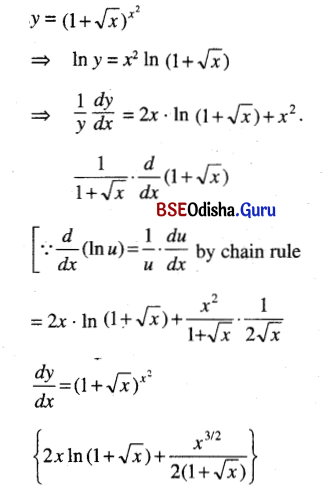Question 7.
$$\left(\sin ^{-1} x\right)^{\sqrt{1-x^2}}$$
Solution: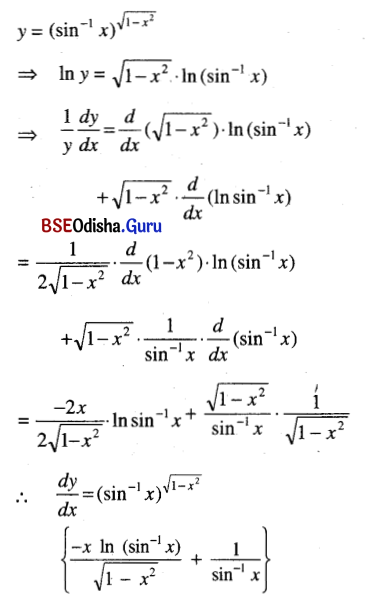Question 8.
$$(\tan x)^{\log x^3}$$
Solution: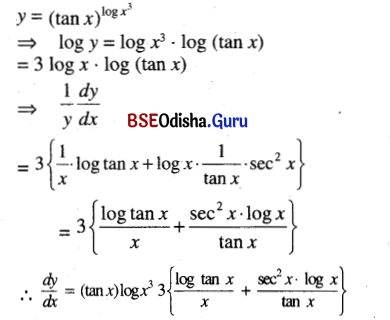Question 9.
x1/x + (sin x)x
Solution: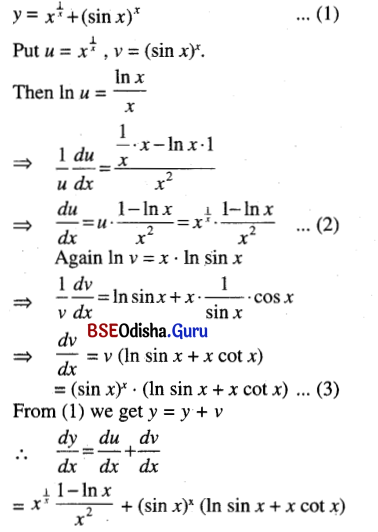Question 10.
(cos x)x + xcos x
Solution: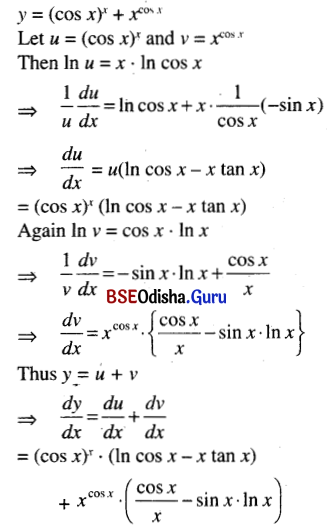Question 11.
(x2 + 1)2/3 (3x + 1)1/4 √x
Solution: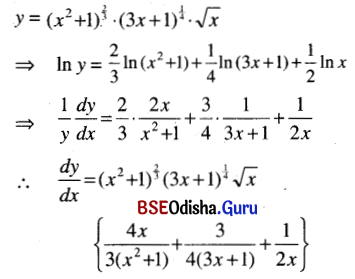Question 12.
$$\frac{(x+1)(x+2)^2(x+3)^3}{(x-1)(x-2)^2(x-3)^3}$$
Solution: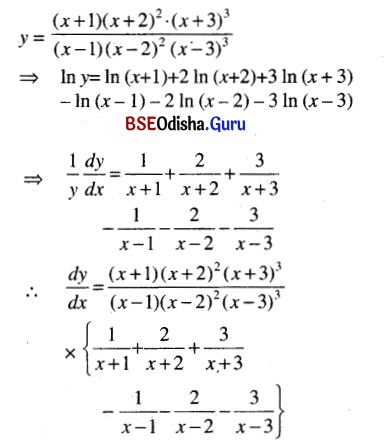Question 13.
(sin x)x $$\sqrt{\sin x}\left(1+x^2\right)^{\frac{1}{2}+x}$$
Solution: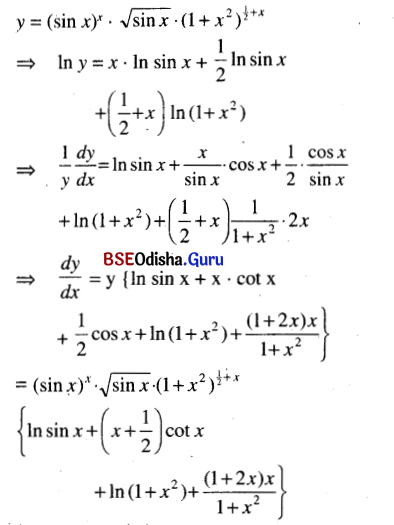Question 14.
(sec x + tan x)cot x
Solution:
y = (sec x + tan x)cot x
⇒ In y = cot x . In (sec x + tan x)
⇒ $$\frac{1}{y}$$$$\frac{d y}{d x}$$ = -cosec2 x . In (sec x + tan x) + cot x . $$\frac{1}{\sec x+\tan x}$$ × (sec x . tan x + sec2 x)
= -cosec2 x . In (sec x + tan x) + cot x . sec x
∴ $$\frac{d y}{d x}$$ = y {-cosec2 x . In (sec x + tan x) + cosec x}
= (sec x + tan x)cot x {cosec x – cosec2 x . In (sec x + tan x)}

Question 15.
(2√x)1+√x
Solution: